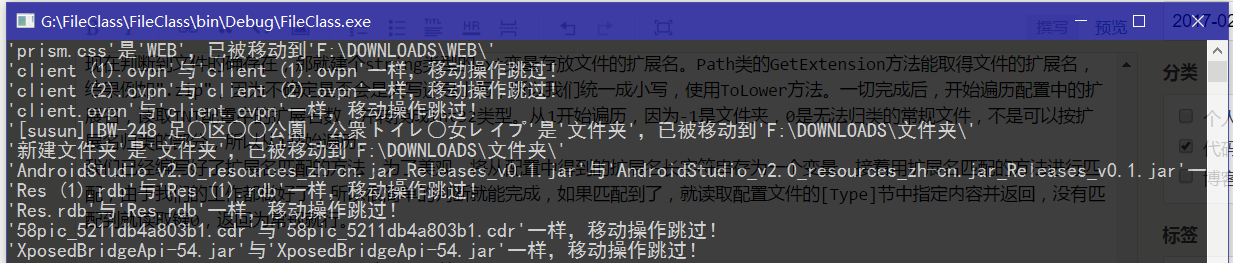# 文件分类程序的编写与原理

@伊吹澪  2017/02/01 14:12

## 准备工作：

C#控制台程序默认的代码是这样的：

``````
using System;
using System.Collections.Generic;
using System.Linq;
using System.Text;

namespace FileClass
{
class Program
{
static void Main(string[] args)
{
}
}
}
``````

Main方法里默认提供了一个string数组类型的args参数，这是外部传入的参数，在命令行输入"fileclass.exe a"，就会在程序中传入一个"a"的参数，参数args数组里的第一个成员就是这个"a"参数，在C#里，数组成员索引以0开始，现在我们明白了传入参数的意义，那么我们就来写个args参数传入的实例。

``````
static void Main(string[] args)
{
foreach(string i in args)
{
Console.WriteLine(i);
}
}
``````

``````
G:\FileClass\FileClass\bin\Debug>fileclass.exe a b c
a
b
c

G:\FileClass\FileClass\bin\Debug>
``````

``````
G:\FileClass\FileClass\bin\Debug\FileClass.pdb
``````

``````
[All]
Num=13

[Type]
-1=文件夹
0=常规
1=应用程序
2=压缩包
3=图片
4=音乐
5=视频
6=种子
7=文档
8=文本文档
9=手机程序
10=镜像
11=脚本
12=WEB
13=字体

[Ext]
1=exe msi
2=zip rar r0* r1* arj gz sit sitx sea ace bz2 7z tar tbz
3=jpg jpeg bmp gif png tga tif dds
4=mp3 wav wma mpa ram ra aac aif m4a ape mid tta
5=avi mpg mpe mpeg asf wmv mov qt rm mp4 flv m4v webm ogv ogg mkv ts swf
6=torrent
7=doc pdf ppt pps docx pptx wps
8=txt ini conf
9=apk ipa
10=iso wim img
11=bat sh py vbs js reg crx nvg bin sp
12=html php jsp
13=ttf ttc
``````

## 开始编写：

``````
///
/// INI操作类
///
public class INI
{
[System.Runtime.InteropServices.DllImport("kernel32")]
private static extern long WritePrivateProfileString(string section, string key, string val, string filePath);
[System.Runtime.InteropServices.DllImport("kernel32")]
private static extern int GetPrivateProfileString(string section, string key, string def, System.Text.StringBuilder retVal, int size, string filePath);
private string sPath = null;
///
/// 设置INI地址
///
/// 地址
public INI(string path)
{
this.sPath = path;
}

///
/// 写入INI
///
/// 节点
/// 键
/// 值
public void WirteINI(string section, string key, string value)
{
WritePrivateProfileString(section, key, value, sPath);
}

///
/// 读取INI
///
/// 节点
/// 键
/// 默认值
///
public string ReadINI(string section, string key,string def="")
{
System.Text.StringBuilder temp = new System.Text.StringBuilder(255);
GetPrivateProfileString(section, key, def, temp, 255, sPath);
return temp.ToString();
}
}
``````

``````
INI Conf=new INI("C://test.ini");
``````

``````
Conf.WriteINI("Test","Test1","Test");
``````

``````
``````

``````
``````

``````
///
/// 扩展名操作类
///
public class Ext
{
///
/// 配置文件
///
private INI Conf;
///
/// 初始化本类
///
/// INI配置路径
public Ext(string conf)
{
Conf = new INI(conf);
}
}
``````

``````
string Conf = Path.GetDirectoryName(Process.GetCurrentProcess().MainModule.FileName) + "\\FileClass.ini";
``````

``````
using System.IO;
using System.Diagnostics;
``````

Path类里有个GetDirectoryName的方法，作用是返回指定路径字符串的目录信息，参数只有一个：文件或目录的路径。Process.GetCurrentProcess().MainModule.FileName是获得程序在进程中的绝对路径，这个和Path.GetCurrentDirectory不一样的是，前者获得的是程序的绝对路径，后者是获得程序的工作路径。

``````
while(File.Exists(Conf)==false)
{
File.WriteAllText(Conf, "[All]\n\n[Type]\n\n[Ext]\n", Encoding.ASCII);
INI tmpini = new INI(Conf);
tmpini.WirteINI("All", "Num", "1");
tmpini.WirteINI("All", "Folder", "");
tmpini.WirteINI("Type", "-1", "文件夹");
tmpini.WirteINI("Type", "0", "常规");
tmpini.WirteINI("Type", "1", "应用程序");
tmpini.WirteINI("Ext", "1", "exe msi");
}
``````

``````
try
{
}
catch(Exception M)
{
Console.WriteLine(M.Message);
}

``````

``````
Ext ExtType = new Ext(Conf);
``````

``````
if (args.Length > 0)
{
}
else
{
Console.WriteLine("没有文件被载入。");
}
``````

Main方法的参数args是个数组，所以需要对args遍历一遍，可以使用foreach语句或for语句，这里我们用for语句：

``````
for (int i = 0; i < args.Length; i++)
{
}
``````

``````
string OPath, NPath,Type;
OPath = args[i];
Type = ExtType.GetType(OPath);
NPath = ExtType.GetFolder() + Type + @"\";
``````

ExtType是我们刚刚实例化的Ext类，GetType和GetFolder是我们自己定义的方法，OPath是文件的旧路径，NPath是文件的新路径，Type是文件类型，这三个变量都是string类型的变量。现在暂停对Main方法的编写，接下来对Ext类进行完善。

``````
///
/// 取得归纳目录路径
///
///
public string GetFolder()
{
}
``````

``````
[Type]
-1=文件夹
2=压缩包
[Ext]
2=zip rar r0* r1* arj gz sit sitx sea ace bz2 7z tar tbz
``````

``````
///
/// 将扩展名配置存为数组
///
/// 扩展名配置
///
private string[] CutExt(string Ext)
{
string[] Exts = Ext.Split(' ');
return Exts;
}
``````

``````
///
/// 判断扩展名是否符合
///
/// 判断的扩展名
/// 被判断的扩展名配置
///
private bool IsExt(string Ext, string Exts)
{
Ext = Ext.Substring(1);
string[] exts = CutExt(Exts);
for (int i = 0; i < exts.Length; i++)
{
string ext = exts[i];
if (ext.IndexOf("*") >= 0)
{
if (Regex.IsMatch(Ext, ConvertRegex(ext)))
{
return true;
}
}
else
{
if (Ext == ext)
{
return true;
}
}
}
return false;
}
``````

``````
///
/// 正则表达式转换
///
/// 待转换字符串
///
public string ConvertRegex(string Str)
{
return "^" + Regex.Escape(Str).Replace("\\*", ".*").Replace("\\?", ".") + "\$";
}
``````

``````
using System.Text.RegularExpressions;
``````

``````

.zip

zip
``````

``````
///
/// 获得指定路径类型
///
/// 文件路径
///
public string GetType(string Name)
{
if (File.Exists(Name))
{
string ext = Path.GetExtension(Name).ToLower();
for (int i = 1; i <= Convert.ToInt32(Conf.ReadINI("All", "Num")); i++)
{
if (IsExt(ext, FileExt))
{
}

}
}
else
{
}
}
``````

File类的Exists方法是判断文件是否存在，文件夹不是文件，当然判断不存在了，不存在它就是文件夹，当然这个不是很严谨，万一真的什么都不存在呢，可以按需完善它。

## 程序核心编写:

``````
if (ExtType.IsSystemFolder(OPath) == false)
{
}
else
{
Console.WriteLine("'{0}'是归纳文件夹，移动操作跳过！", OPath);
}
``````

``````
///
/// 判断是否为归纳文件夹
///
/// 文件夹名
///
public bool IsSystemFolder(string FolderName)
{
for (int i = -1; i <= Convert.ToInt32(Conf.ReadINI("All", "Num")); i++)
{
string FolderType = GetFolder() + Conf.ReadINI("Type", Convert.ToString(i));
if (FolderName == FolderType)
{
return true;
}
}
return false;
}
``````

``````
while (Directory.Exists(NPath) == false)
{
Directory.CreateDirectory(NPath);
Console.WriteLine("'{0}'不存在，已创建该文件夹！", NPath);
}
``````

``````
if (OPath != NPath + Path.GetFileName(OPath))
{
}
else
{
Console.WriteLine("'{0}'与'{1}'一样，移动操作跳过！", Path.GetFileName(OPath), Path.GetFileName(NPath + Path.GetFileName(OPath)));
}
``````

Path类的GetFileName方法是取得某路径的文件名或文件夹名。

``````
if (Type == "文件夹")
{
Directory.Move(OPath, NPath + Path.GetFileName(OPath));
}
else
{
File.Move(OPath, NPath + Path.GetFileName(OPath));
}
Console.WriteLine("'{0}'是'{1}'，已被移动到'{2}'", Path.GetFileName(OPath), Type, NPath);
````````````
startIndex cannot be larger than length of string.
Parameter name: startIndex
``````

## 补充

``````
Ext=Ext.Substring(1);
``````

``````
Ext=Ext.Substring(Ext.IndexOf(".")+1);
``````

### 添加新评论